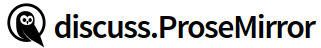# What should be the input rule to convert - - to horizontal rule?

I want to convert ‘- -’ into a horizontal rule. Unable to understand which input rule to use.

You’ll have to write one yourself, passing a function as second argument to the `InputRule` constructor.

Hey recently wrote something similar the code would be something like this:

``````new InputRule(
/^(?:---|___\s|\*\*\*\s)\$/,
(state, match, start, end) => {
if (!match) {
return null;
}
let tr = state.tr.replaceWith(start - 1, end, type.createChecked());
// Find the paragraph that contains the "---" shortcut text, we need
// it below for deciding whether to insert a new paragraph after the
// hr.
const \$para = state.doc.resolve(start);

let insertParaAfter = false;
if (\$para.end() != end) {
// if the paragraph has more characters, e.g. "---abc", then no
// need to insert a new paragraph
insertParaAfter = false;
} else if (\$para.after() == \$para.end(-1)) {
// if the paragraph is the last child of its parent, then insert a
// new paragraph
insertParaAfter = true;
} else {
const nextNode = state.doc.resolve(\$para.after()).nodeAfter!;
// if the next node is a hr, then insert a new paragraph
insertParaAfter = nextNode.type === type;
}
return insertParaAfter
? safeInsert(
state.schema.nodes.paragraph.createChecked(),
tr.mapping.map(\$para.after()),
)(tr)
: tr;
},
)
``````